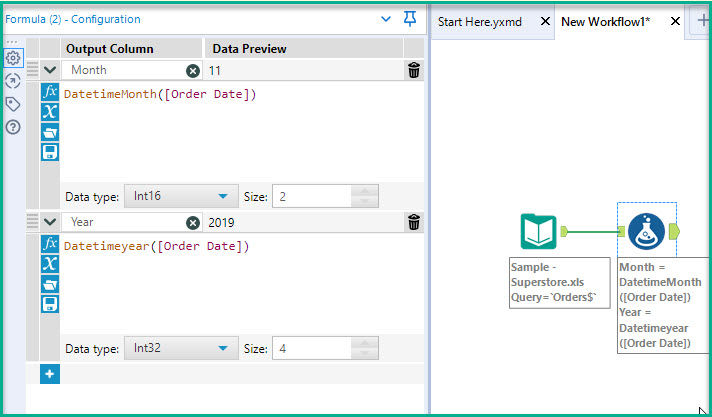top of page

# Multi-Row Formula Tool - Calculating a Moving 12 Months

Do you need to calculate a total value for the last 12 months? If you have data from January - December, you can use the Summarize Tool to get the total for a full year's worth of data. But what if its March and you want to calculate the total for this length of time? (Example: February 2021 - March 2022) use the Multi-Row Formula Tool!

In the workflow below I am calculating the total sales for each month, using the Sample Superstore data set that is packaged with the Tableau application.

First, I create a column for both Month and Year to extract these values as a new column in my data.Next, I use the Summarize Tool to pull the Total Sales for Month and Year.Next, I add the Multi-Row Formula Tool. I select the option to create a New Field and enter Running Year Total as the custom name for this column. I enter 12 as the number of Rows I want to count back.

Alteryx will automatically add Row - 1, Row - 2, etc.The following expression is used to add together 11 previous rows with the current row for the Sales column. This will be the moving calculation for Sales.In the Results Pane you can see the total for row 12 is the same amount you would receive if you used the Summarize Tool, if you were to Sum by Year.The next row 13 is calculating the sum for January 2018 all the way back to February 2017. Its the equivalent of (4519.892+55619.009+28295.345+23648.287+34595.1276+33946.393+27909.4685+

81777.3508+31453.393+78628.7167+69545.6205+18174.0756)

Row 14 is calculating the sum for February 2018 back to March 2017 and so on.Here is what the data would look like if you used a Sum formula to do the same calculation in Excel;*You can also use the Multi-Row Formula Tool to obtain a moving average for 12 months.